Function Repository Resource:

AntidiagonalMatrix

Creates an antidiagonal matrix by given the antidiagonal

Contributed by: Sander Huisman
 ResourceFunction["AntidiagonalMatrix"][list] gives a matrix with the elements of list on the leading antidiagonal, and 0 elsewhere. ResourceFunction["AntidiagonalMatrix"][list,k] gives a matrix with the elements of list on the kth antidiagonal. ResourceFunction["AntidiagonalMatrix"][list,k,n] pads with 0s to create an n×n matrix.

Details and Options

ResourceFunction["AntidiagonalMatrix"][list] creates a matrix where the antidiagonal is given by the elements xi of list: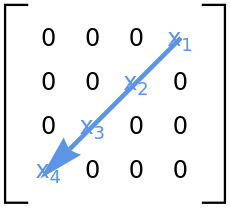For positive k, ResourceFunction["AntidiagonalMatrix"][list,k] puts the elements k positions above the main antidiagonal. ResourceFunction["AntidiagonalMatrix"][list,-k] puts the elements k positions below.
AntidiagonalMatrix[list,k] fills the kth antidiagonal of a square matrix with the elements from list. Different values of k lead to different matrix dimensions.
ResourceFunction["AntidiagonalMatrix"][list,k,n] always creates an n×n matrix, even if this requires dropping elements of list.
AntidiagonalMatrix[list,k,{m,n}] creates an m×n matrix.
ResourceFunction["AntidiagonalMatrix"][SparseArray[…],…] gives a SparseArray object.

Examples

Basic Examples (3)

Construct an antidiagonal matrix:

 In:=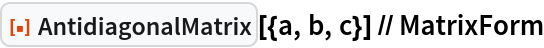Out=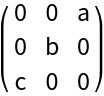A super-antidiagonal matrix:

 In:=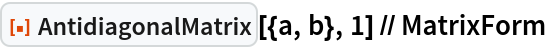Out=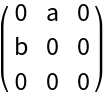A sub-antidiagonal matrix:

 In:=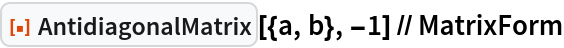Out=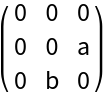Scope (4)

The elements in AntidiagonalMatrix are chosen to match the elements of the vector:

 In:=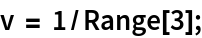Exact number entries:

 In:=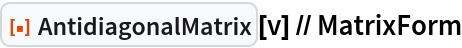Out=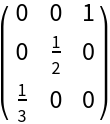Machine-number entries:

 In:=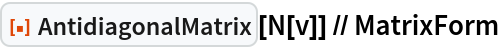Out=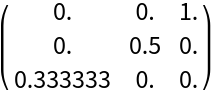Arbitrary-precision number entries:

 In:=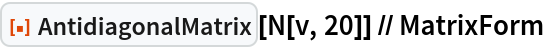Out=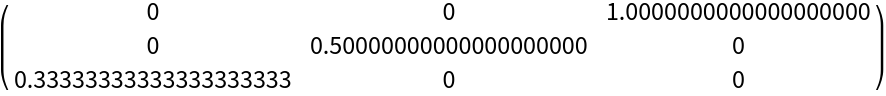When the vector is a SparseArray object, AntidiagonalMatrix will give a SparseArray object:

 In:=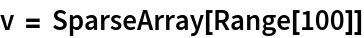Out=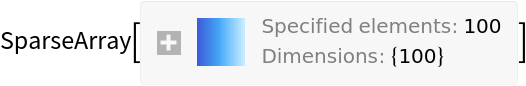In:=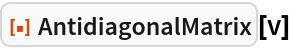Out=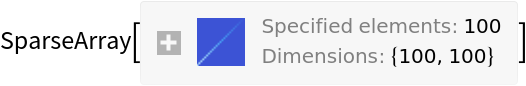Pad with zeros to make a larger square matrix:

 In:=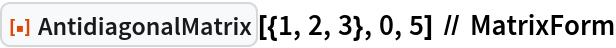Out=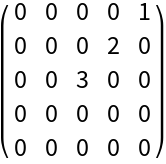Make a square matrix with the specified dimension:

 In:=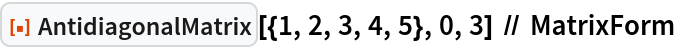Out=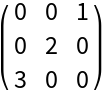Rectangular diagonal matrices:

 In:=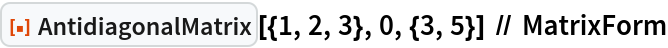Out=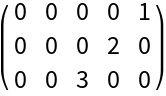In:=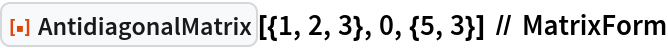Out=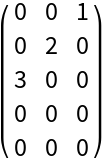Applications (3)

Express a matrix as the sum of its antidiagonal and off-antidiagonal parts:

 In:=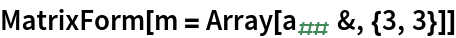Out=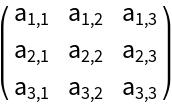In:=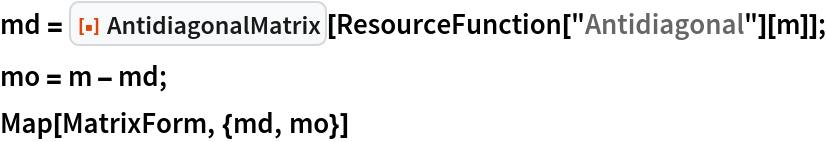Out=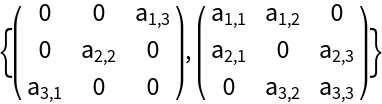Construct a 5×5 tri-antidiagonal matrix:

 In:=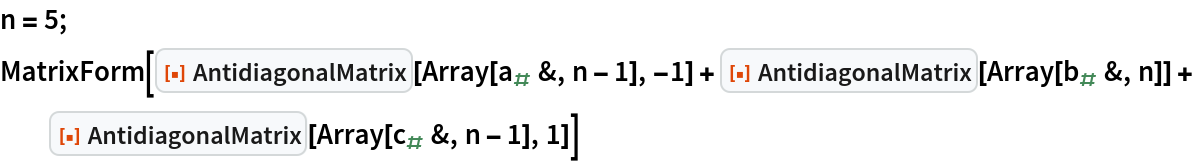Out=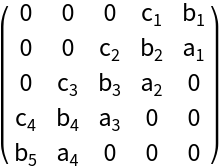Extract the antidiagonal from an antidiagonal rectangular matrix:

 In:=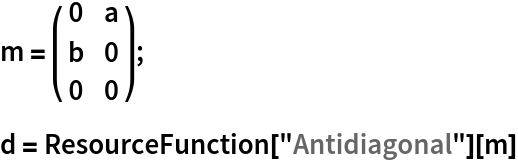Out=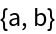Reconstruct the original matrix from the antidiagonal:

 In:=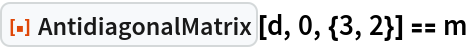Out=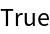Properties and Relations (1)

Using the resource function Antidiagonal with AntidiagonalMatrix gives the original vector:

 In:=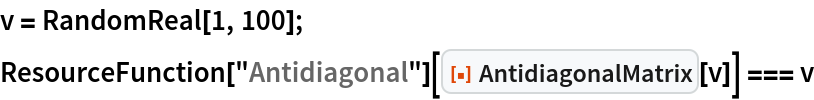Out=